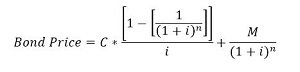# You have just purchased a 10-year, $1,000 par value bond, The coupon rate is 8% annually, with... ## Question: You have just purchased a 10-year,$1,000 par value bond, The coupon rate is 8% annually, with interest being paid each 6 months.

If you expect to earn a 10% nominal rate of return on this bond, how much did you pay for it

a. 1,122.87

b. 1,003.42

c. 875.38

d. 950.75

e. 812.15

## Answer and Explanation:

The correct choice is Option C.

Bond Price is given as:Here,

M = Maturity Amount = $1,000.00 n = Number of periods to maturity = 10 x 2 = 20 C = Periodic coupon = 1000 x 8% x 1/2 =$40.00

i = Periodic yield = 10 / 2 = 5% = 0.05

Thus,

Bond Price = \$875.38